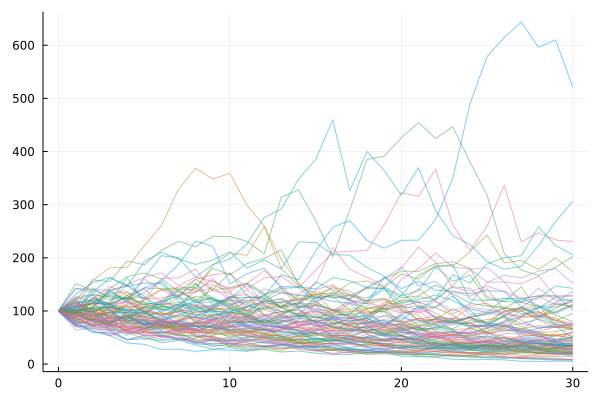## EconomicScenarioGenerators.jl

Status: Technical Preview
Author JuliaActuary
Popularity
15 Stars
Updated Last
9 Months Ago
Started In
May 2022

# EconomicScenarioGenerators.jl

Help wanted!

This package is in Technical Preview Stage: The API is stabilizing and tests are passing but it has not been used in practice for very long. Please report any issues, provide feedback, and request specific features using the Discussions or Issues in this repository.

Interested in developing economic scenario generators in Julia? Consider contributing to this package. Open an issue, create a pull request, or discuss on the Julia Zulip's #actuary channel.

## Usage

EconoicScenarioGenerators.jl is now available via the General Registry. Install and use in the normal way:

2. `import EconomicScenarioGenerators` or `using EconomicScenarioGenerators` in your code

## Examples

### Importing packages

First, import both `EconomicScenarioGenerators` and `Yields`:

``````using EconomicScenarioGenerators
using Yields
``````

## Models

### Interest Rate Models

• `Vasicek`
• `CoxIngersolRoss`
• `HullWhite`

### EquityModels

• `BlackScholesMerton`

### Interest Rate Model Examples

#### Vasicek

```m = Vasicek(0.136,0.0168,0.0119,Continuous(0.01)) # a, b, σ, initial Rate
s = ScenarioGenerator(
1,  # timestep
30, # projection horizon
m,  # model
)```

This can be iterated over, or you can collect all of the rates like:

`rates = collect(s)`

or

```for r in s
# do something with r
end```

And the package integrates with Yields.jl:

```YieldCurve(s)
```

will produce a yield curve object:

``````              ⠀⠀⠀⠀⠀⠀⠀⠀⠀⠀⠀⠀⠀⠀Yield Curve (Yields.BootstrapCurve)⠀⠀⠀⠀⠀⠀⠀⠀⠀⠀⠀⠀⠀
┌────────────────────────────────────────────────────────────┐
0.03 │⠀⠀⠀⠀⠀⠀⠀⠀⠀⠀⠀⠀⠀⠀⠀⠀⠀⠀⠀⠀⠀⠀⠀⠀⠀⠀⠀⠀⠀⠀⠀⠀⠀⠀⠀⠀⠀⠀⠀⠀⠀⠀⠀⠀⠀⣀⠤⠤⠔⠒⠉⠉⠒⠒⠒⠒⠒⠤⣄⣀│ Zero rates
│⠀⠀⠀⠀⠀⠀⠀⠀⠀⠀⠀⠀⠀⠀⠀⠀⠀⠀⠀⠀⠀⠀⠀⠀⠀⠀⠀⠀⠀⠀⠀⠀⠀⠀⠀⠀⠀⠀⠀⠀⣀⠤⠒⠒⠉⠀⠀⠀⠀⠀⠀⠀⠀⠀⠀⠀⠀⠀⠀⠀│
│⠀⠀⠀⠀⠀⠀⠀⠀⠀⠀⠀⠀⠀⠀⠀⠀⠀⠀⠀⠀⠀⠀⠀⠀⠀⠀⠀⠀⠀⠀⠀⠀⠀⠀⠀⠀⠀⣀⠔⠊⠁⠀⠀⠀⠀⠀⠀⠀⠀⠀⠀⠀⠀⠀⠀⠀⠀⠀⠀⠀│
│⠀⠀⠀⠀⠀⠀⠀⠀⠀⠀⠀⠀⠀⠀⠀⠀⠀⠀⠀⠀⠀⠀⠀⠀⠀⠀⠀⠀⠀⠀⠀⠀⠀⠀⣀⠔⠋⠁⠀⠀⠀⠀⠀⠀⠀⠀⠀⠀⠀⠀⠀⠀⠀⠀⠀⠀⠀⠀⠀⠀│
│⠀⠀⠀⠀⠀⠀⠀⠀⠀⠀⠀⠀⠀⠀⠀⠀⠀⠀⠀⠀⠀⠀⠀⠀⢀⣀⣀⠀⠀⣀⡤⠖⠊⠉⠀⠀⠀⠀⠀⠀⠀⠀⠀⠀⠀⠀⠀⠀⠀⠀⠀⠀⠀⠀⠀⠀⠀⠀⠀⠀│
│⠀⠀⠀⠀⠀⠀⠀⠀⠀⠀⠀⠀⠀⠀⠀⠀⠀⠀⠀⠀⣀⠤⠖⠋⠁⠀⠀⠉⠉⠁⠀⠀⠀⠀⠀⠀⠀⠀⠀⠀⠀⠀⠀⠀⠀⠀⠀⠀⠀⠀⠀⠀⠀⠀⠀⠀⠀⠀⠀⠀│
│⠀⠀⠀⠀⠀⠀⠀⠀⠀⠀⠀⠀⠀⠀⠀⠀⠀⣀⠔⠋⠀⠀⠀⠀⠀⠀⠀⠀⠀⠀⠀⠀⠀⠀⠀⠀⠀⠀⠀⠀⠀⠀⠀⠀⠀⠀⠀⠀⠀⠀⠀⠀⠀⠀⠀⠀⠀⠀⠀⠀│
Continuous │⠀⠀⠀⠀⠀⠀⠀⠀⠀⣀⡤⠒⠓⠦⠤⠖⠉⠀⠀⠀⠀⠀⠀⠀⠀⠀⠀⠀⠀⠀⠀⠀⠀⠀⠀⠀⠀⠀⠀⠀⠀⠀⠀⠀⠀⠀⠀⠀⠀⠀⠀⠀⠀⠀⠀⠀⠀⠀⠀⠀│
│⠀⠀⠀⠀⠀⠀⠀⢰⠋⠁⠀⠀⠀⠀⠀⠀⠀⠀⠀⠀⠀⠀⠀⠀⠀⠀⠀⠀⠀⠀⠀⠀⠀⠀⠀⠀⠀⠀⠀⠀⠀⠀⠀⠀⠀⠀⠀⠀⠀⠀⠀⠀⠀⠀⠀⠀⠀⠀⠀⠀│
│⠀⠀⣀⠖⠢⡀⡰⠃⠀⠀⠀⠀⠀⠀⠀⠀⠀⠀⠀⠀⠀⠀⠀⠀⠀⠀⠀⠀⠀⠀⠀⠀⠀⠀⠀⠀⠀⠀⠀⠀⠀⠀⠀⠀⠀⠀⠀⠀⠀⠀⠀⠀⠀⠀⠀⠀⠀⠀⠀⠀│
│⠉⠉⠁⠀⠀⠉⠁⠀⠀⠀⠀⠀⠀⠀⠀⠀⠀⠀⠀⠀⠀⠀⠀⠀⠀⠀⠀⠀⠀⠀⠀⠀⠀⠀⠀⠀⠀⠀⠀⠀⠀⠀⠀⠀⠀⠀⠀⠀⠀⠀⠀⠀⠀⠀⠀⠀⠀⠀⠀⠀│
│⠀⠀⠀⠀⠀⠀⠀⠀⠀⠀⠀⠀⠀⠀⠀⠀⠀⠀⠀⠀⠀⠀⠀⠀⠀⠀⠀⠀⠀⠀⠀⠀⠀⠀⠀⠀⠀⠀⠀⠀⠀⠀⠀⠀⠀⠀⠀⠀⠀⠀⠀⠀⠀⠀⠀⠀⠀⠀⠀⠀│
│⠀⠀⠀⠀⠀⠀⠀⠀⠀⠀⠀⠀⠀⠀⠀⠀⠀⠀⠀⠀⠀⠀⠀⠀⠀⠀⠀⠀⠀⠀⠀⠀⠀⠀⠀⠀⠀⠀⠀⠀⠀⠀⠀⠀⠀⠀⠀⠀⠀⠀⠀⠀⠀⠀⠀⠀⠀⠀⠀⠀│
│⠀⠀⠀⠀⠀⠀⠀⠀⠀⠀⠀⠀⠀⠀⠀⠀⠀⠀⠀⠀⠀⠀⠀⠀⠀⠀⠀⠀⠀⠀⠀⠀⠀⠀⠀⠀⠀⠀⠀⠀⠀⠀⠀⠀⠀⠀⠀⠀⠀⠀⠀⠀⠀⠀⠀⠀⠀⠀⠀⠀│
0 │⠀⠀⠀⠀⠀⠀⠀⠀⠀⠀⠀⠀⠀⠀⠀⠀⠀⠀⠀⠀⠀⠀⠀⠀⠀⠀⠀⠀⠀⠀⠀⠀⠀⠀⠀⠀⠀⠀⠀⠀⠀⠀⠀⠀⠀⠀⠀⠀⠀⠀⠀⠀⠀⠀⠀⠀⠀⠀⠀⠀│
└────────────────────────────────────────────────────────────┘
⠀0⠀⠀⠀⠀⠀⠀⠀⠀⠀⠀⠀⠀⠀⠀⠀⠀⠀⠀⠀⠀⠀⠀⠀⠀⠀⠀time⠀⠀⠀⠀⠀⠀⠀⠀⠀⠀⠀⠀⠀⠀⠀⠀⠀⠀⠀⠀⠀⠀⠀⠀⠀⠀⠀30⠀
``````

#### CoxIngersolRoss

A CIR model:

`m = CoxIngersollRoss(0.136,0.0168,0.0119,Continuous(0.01))`

#### Hull White Model using a Yields.jl YieldCurve

Construct a yield curve and use that as the arbitrage-free forward curve within the Hull-White model.

```using Yields, EconomicScenarioGenerators
rates =[0.01, 0.01, 0.03, 0.05, 0.07, 0.16, 0.35, 0.92, 1.40, 1.74, 2.31, 2.41] ./ 100
mats = [1/12, 2/12, 3/12, 6/12, 1, 2, 3, 5, 7, 10, 20, 30]
c = Yields.CMT(rates,mats)

m = HullWhite(.1,.002,c) # a, σ, curve

s = ScenarioGenerator(
1/12,  # timestep
30., # projection horizon
m,  # model
)```

Create 1000 yield curves from the scenario generator:

```n = 1000
curves = [YieldCurve(s) for i in 1:n]
```julia

Plot the result:

```julia
using Plots

times = 1:30
p=plot(title="EconomicScenarioGenerators.jl Hull White Model")
for d in curves
plot!(p,times,Yields.rate.(Yields.zero.(d,times)),alpha=0.2,label="")
end

plot!(times,Yields.rate.(Yields.zero.(c,times)),line=(:black, 5), label="Given Yield Curve")
p
```### Equity Model Examples

#### BlackScholesMerton

```m = BlackScholesMerton(0.01,0.02,.15,100.)

s = ScenarioGenerator(
1,  # timestep
30, # projection horizon
m,  # model
)```

Instantiate an array of the projection with `collect(s)`.

##### Plotted BSM Example

Plot 100 paths:

``````using Plots
projections = [collect(s) for _ in 1:100]

p = plot()

for p in projections
plot!(0:30,p,label="",alpha=0.5)
end

p
``````## Correlated Scenarios

Combined with `using Copulas`, you can create correlated scenarios with a given copula. See `?Correlated` for the docstring on creating a correlated set of scenario generators.

### Example

Create two equity paths that are 90% correlated:

``````using EconomicScenarioGenerators, Copulas

m = BlackScholesMerton(0.01,0.02,.15,100.)
s = ScenarioGenerator(
1,  # timestep
30, # projection horizon
m,  # model
)

ss = [s,s] # these don't have to be the exact same, but do need same shape
g = ClaytonCopula(2,7) # highly dependendant model
c = Correlated(ss,g)

collect(c)

using Plots
plot(collect(c))
``````## Benchmarks

Generating 10,000 scenarios of daily timesteps for 1 year (252 business days) with a Black-Scholes-Merton model:

library multi-threaded? pre-allocated arrays? language time (absolute) time (relative)
EconomicScenarioGenerators 8x Yes Julia 5ms 1x
EconomicScenarioGenerators 8x No Julia 6ms 1x
EconomicScenarioGenerators No Yes Julia 19ms 4x
EconomicScenarioGenerators No No Julia 20ms 4x
pyesg No? No Python 135ms 27x

## Other ESG packages

### Required Packages

View all packages

### Used By Packages

No packages found.